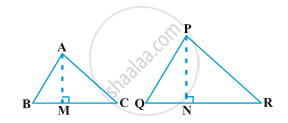# Areas of Similar Triangles

#### Topics

• Theorem: When two triangles are similar, the ratio of areas of those triangles is equal to the ratio of the squares of their corresponding sides.

## Theorem

Theorem1: The ratio of the areas of two similar triangles is equal to the square of the ratio of their corresponding sides.Given: ∆ABC ∼ ∆PQR
Construction: Draw AM ⊥ BC and PN ⊥ QR

To prove: "ar∆ABC"/"ar∆PQR" = ("AB"/"PQ")^2= ("BC"/"QR")^2= ("AC"/"PR")^2

Proof: ∆ABC ∼ ∆PQR (Given)

"AB"/"PQ"= "BC"/"QR" = "AC"/"PR" (Corresponding parts of Congruent triangles) ...eq1

and ∠B= ∠Q (Corresponding parts of Congruent triangles)

Now, "ar(∆ABC)"= 1/2 × "BC" × "AM"

"ar(∆PQR)"= 1/2 × "QR" × "PN"

"ar(∆ABC)"/"ar(∆PQR)"= (1/2 xx "BC" xx "AM")/(1/2 xx "QR" xx "PN")

"ar(∆ABC)"/"ar(∆PQR)"= "BC"/"QR"×"AM"/"PN" .......eq2

Again, In ∆ABM and ∆PQN
∠AMB = ∠PNQ = 90°
∠B= ∠Q (Corresponding parts of Congruent triangles)
∆ABM ∼ ∆PQN

So, "AB"/"PQ"= "AM"/"PN" (Corresponding parts of Congruent triangles) .....eq3

From eq2 and eq3

"ar(∆ABC)"/"ar(∆PQR)"= "BC"/"QR"×"AB"/"PQ" ......eq4

From eq1 and eq4

"ar(∆ABC)"/"ar(∆PQR)"= "BC"/"QR" × "BC"/"QR"

"ar(∆ABC)"/"ar(∆PQR)"= ("BC"/"QR")^2 .........eq5

From eq1 and eq5

"ar(∆ABC)"/"ar(∆PQR)"= ("AB"/"PQ")^2 = ("BC"/"QR")^2 = ("AC"/"PR")^2

Hence proved.

If you would like to contribute notes or other learning material, please submit them using the button below.

### Shaalaa.com

Triangles part 37 (Example Ratio of Area of triangle) [00:10:05]
S
0%